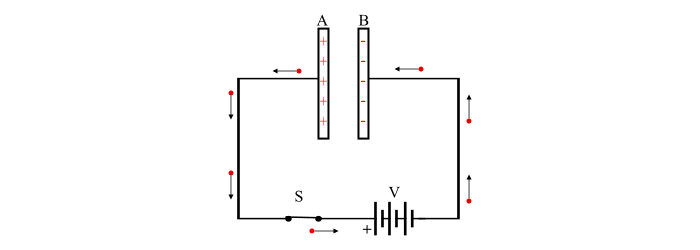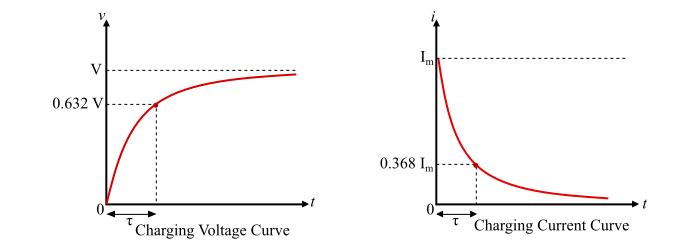# Capacitors in DC Circuits

## Capacitor & Capacitance

When any two conducting surfaces are separated by an insulating material, it called as a capacitor. The conducting surfaces are known as plates of the capacitor and the insulating material is known as dielectric.

The ability of a capacitor to store charge is termed as capacitance of the capacitor. It is denoted by C and measured in Farad (F).

By experiments, it has been found that charge (Q) stored in a capacitor is directly proportional to the potential difference across it, i.e.,

$$Q\varpropto\:V$$

$$\Rightarrow\:Q=CV$$

$$\Rightarrow\:C=\frac{Q}{V}\:\:\:\:...(1)$$

Where, C is a constant, known as capacitance of the capacitor.

Hence, the capacitance (C) of a capacitor is defined as the ratio of charge (Q) on either plate of the capacitor to the potential difference (V) across it.

The unit of capacitance is

$$\because\:C=\frac{Q}{V}$$

$$\therefore\:Unit\:of\:C=\frac{Coulomb}{Volt}=Farad$$

## How does a capacitor store charge?

Consider a parallel plate capacitor connected across a battery of V volts through a switch. The charging of a capacitor can be understood as follows −

Step 1 − With the switch S open, there is no charge on the plates of the capacitor.Step 2 − When the switch S is closed, the positive terminal of the battery attracts the electrons from plate A and accumulate these electrons on to the plate B. This results in that, the plate A becomes more and more positive and the plate B becomes more and more negative. This action is referred to as charging of a capacitor. This process of charging of capacitor continues till potential difference across the capacitor becomes equal to the battery voltage (V).Step 3 − As soon as, the capacitor is charged a battery voltage (V), the current flow stops.Step 4 – Now, if the switch S is opened, the capacitor plates will retain the charge. Therefore, at this time, the capacitor is said to be charged.## Important Points

The following point may be noted about the action of capacitor −

• When a DC voltage is applied across a capacitor, a charging current will flow until the capacitor is fully charged when the current is stopped. This charging process will take place in a very short time, a fraction of a second. Hence, a fully charged capacitor blocks the flow of DC current.

• There is only a transfer of electrons from one plate to the other through the external circuit. The current does not flow in between the plates of the capacitor.

• When a capacitor is charged, the two plates carry equal and opposite charge. Thus, charge on a capacitor means charge on either plate.

• The energy required to charge a capacitor is supplied by the external source.

## Behaviour of Capacitor in DC Circuit

The behaviour of a capacitor in DC circuit can be understood from the following points −

• When a DC voltage is applied across an uncharged capacitor, the capacitor is quickly (not instantaneously) charged to the applied voltage. The charging current is given by,

$$i=\frac{dQ}{dt}=\frac{d(CV)}{dt}=C\frac{dV}{dt}\:\:\:\:(2)$$

• When the capacitor is fully charged, the voltage across the capacitor becomes constant and is equal to the applied voltage. Therefore, (dV/dt = 0) and thus, the charging current.

• The voltage across an uncharged capacitor is zero, thus it is equivalent to a short circuit as far as DC voltage is concerned.

• When the capacitor is fully charged, there is no current flows in the circuit. Hence, a fully charged capacitor appears as an open circuit to dc.

## Charging of Capacitor

Consider an uncharged capacitor of capacitance C connected across a battery of V volts (D.C.) through a series resistor R to limit the charging current within a safe limit. When the switch S is closed, a charging current flows in the circuit and the capacitor starts to charge.The charging current is maximum at the instant of switch is closed and decreasing gradually as the voltage across the capacitor increases. When the capacitor is fully charged to applied voltage (V), the charging current is reduced to zero.

At the instant when switch is closed

At the instant when the switch is closed, the voltage across the capacitor is zero (as the capacitor is uncharged at the start). The whole voltage V is appeared across resistor R and charging current is maximum. Therefore,

$$Initial\:charging\:current,I_{m}=\frac{V}{R}$$

$$Voltage\:accross\:capacitor,v=0$$

$$Charge\:on\:capacitor,Q=0$$

At any time instant, t

After having closed the switch, the charging current starts decreasing and the voltage across capacitor gradually increases. Therefore, at any time instant t,

$$voltage\:across\:capacitor=v$$

$$charge\:on\:capacitor,q=Cv$$

$$The\:charging\:current,i=C\frac{dv}{dt}$$

Voltage across capacitor

By applying KCL in the circuit, we can write,

$$V=V_{R}+v$$

$$\Rightarrow\:V=iR+v=(C\frac{dv}{dt})R+v\:\:\:\:\:...(3)$$

$$\Rightarrow\:\frac{dv}{V-v}=\frac{dt}{RC}$$

Taking integration on both sides,

$$\int\frac{dv}{V-v}=\int\frac{dt}{RC}$$

On solving this integration, we get,

$$-\log_{e}{(V-v)}=\frac{t}{RC}+K\:\:\:\:...(4)$$

The value of K can be determined from the initial conditions. At the instant of closing the switch, t = 0 and v = 0. Therefore, from the equation (4),

$$-\log_{e}{V}=K$$

Substitute the value of K in the eq. (4), we obtain,

$$-\log_{e}{(V-v)}=\frac{t}{RC}-\log_{e}{(V)}$$

$$\Rightarrow\:\log_{e}{(V-v)}-\log_{e}{(V)}=-\frac{t}{RC}$$

$$\Rightarrow\:\log_{e}{(\frac{V-v}{V})}=-\frac{t}{RC}$$

Taking antilog on both sides, we obtain,

$$\frac{V-v}{V}=e^{-t/RC}$$

$$\Rightarrow\:V-v=Ve^{-t/RC}\:\:\:\:...(5)$$

$$\Rightarrow\:v=V(1-e^{-t/RC})\:\:\:\:...(6)$$

The eq. (5) shows that during the charging, the voltage across the capacitor increases exponentially.

Charging current

From eq. (3),

$$V-v=iR$$

And from eq. (5),

$$V-v=Ve^{-t/RC}$$

$$\therefore\:iR=Ve^{-t/RC}$$

$$\Rightarrow\:i=\frac{V}{R}e^{-t/RC}=I_{m}e^{-t/RC}\:\:\:\:...(7)$$

Where, Im is the initial charging current. Also, from the eq. (7), it can be seen that the charging current reduces exponentially. The equations of charging voltage and charging current can also be represented graphically as shown below.Time Constant

The time constant can be defined as the time required for the capacitor voltage (v) to rise to its final steady value V. It is denoted by Tau (τ) and given by,

$$Time\:Constant,\tau=RC\:seconds\:\:\:\:...(8)$$

## Discharging of Capacitor

Consider a charged capacitor of C farad connected in series with a resistor R through a switch S. When the switch is open the voltage across the capacitor is V volts. When the switch is closed, a discharging current starts to flow in the circuit and the capacitor starts to discharge i.e. voltage across it starts decreasing. The discharging current rises instantaneously to a value Im and then reduces to zero.Discharging voltage

Consider at any time instant t during discharging,

$$Voltage\:across\:capacitor=v$$

$$Discharging\:current,i=C\frac{dV}{dt}$$

By apply KVL in the circuit, we get,

$$V+iR=0$$

$$\Rightarrow\:v+CR\frac{dV}{cdt}=0$$

$$\Rightarrow\:\frac{dV}{v}=-\frac{dt}{RC}$$

Integrating on both sides, we obtain,

$$\log_{e}{v}=-\frac{t}{RC}+K\:\:\:\:...(9)$$

The value of K can be determined from the initial conditions. At the instant of closing the switch, t = 0 and v = V. Thus, from eq. (9),

$$\log_{e}{V}=K$$

Therefore, the eq. (9) becomes,

$$\log_{e}{v}=-\frac{t}{RC}+\log_{e}{V}$$

$$\Rightarrow\log_{e}{\frac{v}{V}}=-\frac{t}{RC}$$

Taking antilog on both the sides, we get,

$${\frac{v}{V}}=e^{-t/RC}$$

$$\Rightarrow\:v=Ve^{-t/RC}\:\:\:\:...(10)$$

The eq. (10) shows that, during the discharging of a capacitor, the voltage across it decreases following exponential law.

Discharging Current

The discharging current flows in the opposite direction to that in which the charging current flows, i.e.

$$i=-I_{m}e^{-t/RC}\:\:\:...(11)$$

The equations of discharging voltage and discharging current can also be represented graphically as shown below.## Numerical Example

A 4 μF capacitor is connected to a DC supply of 120 volts through a resistance of 1 MΩ.

Determine the following −

• Time constant

• Initial charging current

• Voltage across the capacitor 5 seconds after the switch has been closed

• Time taken for the capacitor to fully charged.

Solution

• Time Constant

$$\tau=RC=(1\times\:10^{6})\times\:(4\times\:10^{-6})=4\:sec$$

• Initial charging current

$$I_{m}=\frac{V}{R}=\frac{120}{1\times\:10^{6}}=120\mu\:A$$

• Voltage across the capacitor 5 seconds after the switch has been closed

$$\because\:v=V(1-e^{-t/RC})=120\times\:(1-e^{-5/4})=85.62V$$

• Time taken for the capacitor to fully charged

Time taken for the capacitor to be fully charged = 5 × Time Constant

$$\therefore\:t_{full\:charged}=5\times\:4=20\:sec$$